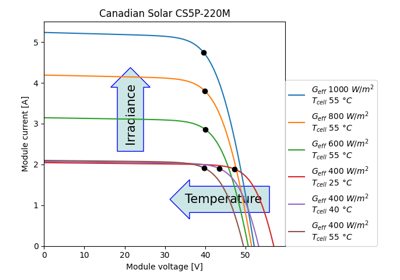# pvlib.pvsystem.i_from_v#

pvlib.pvsystem.i_from_v(voltage, photocurrent, saturation_current, resistance_series, resistance_shunt, nNsVth, method='lambertw')[source]#

Device current at the given device voltage for the single diode model.

Uses the single diode model (SDM) as described in, e.g., Jain and Kapoor 2004 1. The solution is per Eq 2 of  except when resistance_series=0, in which case the explict solution for current is used. Ideal device parameters are specified by resistance_shunt=np.inf and resistance_series=0. Inputs to this function can include scalars and pandas.Series, but it is the caller’s responsibility to ensure that the arguments are all float64 and within the proper ranges.

Changed in version 0.10.0: The function’s arguments have been reordered.

Parameters
• voltage (numeric) – The voltage in Volts under desired IV curve conditions.

• photocurrent (numeric) – Light-generated current (photocurrent) in amperes under desired IV curve conditions. Often abbreviated `I_L`. 0 <= photocurrent

• saturation_current (numeric) – Diode saturation current in amperes under desired IV curve conditions. Often abbreviated `I_0`. 0 < saturation_current

• resistance_series (numeric) – Series resistance in ohms under desired IV curve conditions. Often abbreviated `Rs`. 0 <= resistance_series < numpy.inf

• resistance_shunt (numeric) – Shunt resistance in ohms under desired IV curve conditions. Often abbreviated `Rsh`. 0 < resistance_shunt <= numpy.inf

• nNsVth (numeric) – The product of three components. 1) The usual diode ideal factor (n), 2) the number of cells in series (Ns), and 3) the cell thermal voltage under the desired IV curve conditions (Vth). The thermal voltage of the cell (in volts) may be calculated as `k*temp_cell/q`, where k is Boltzmann’s constant (J/K), temp_cell is the temperature of the p-n junction in Kelvin, and q is the charge of an electron (coulombs). 0 < nNsVth

• method (str) – Method to use: `'lambertw'`, `'newton'`, or `'brentq'`. Note: `'brentq'` is limited to 1st quadrant only.

Returns

current (np.ndarray or scalar)

References

1

A. Jain, A. Kapoor, “Exact analytical solutions of the parameters of real solar cells using Lambert W-function”, Solar Energy Materials and Solar Cells, 81 (2004) 269-277.

## Examples using `pvlib.pvsystem.i_from_v`#Calculating a module’s IV curves

Calculating a module's IV curves